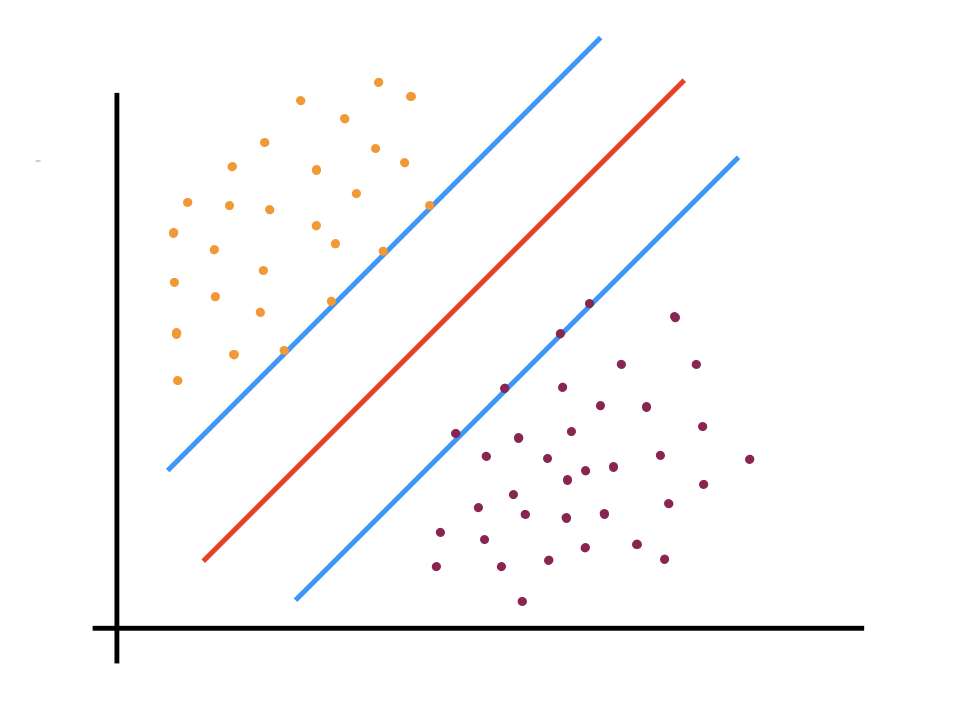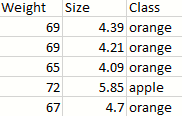1602882000

# Support Vector Machines (SVM)

### History

This article is about an introduction to SVMs, understanding the mathematical intuition, Regularization, implementing the concept in code, and then knowing the fields of its applications. Right then tighten your seat belt and let’s enter this wonderland of beautiful concept Support Vector Machines (SVM). Vladimir Vapnik one of the pioneers in the Machine Learning world had discovered the SVMs in the early 1960s. But he was unable to prove the magic of this Algorithm computationally as he was lacking the needed resources back then in the 1960s. It was in the early 1990s that the Bell Laboratory invited him to come forward to the US related to the research work. Back then in 1993, there was quite a fast track research going on in the character digit recognition area. Vapnik bet that his work of SVMs could do much better than the neural nets in digit recognition. So one of my colleagues tried to apply the SVM and it worked wonders to his surprise. So finally the work which was discovered in the 1960s got recognition in the 1990s when it was actually implemented to give better computational results.

### Introduction

Support Vector Machines is a supervised machine learning algorithm used in Classification and Regression modeling. The purpose of SVMs is to identify a sample in space and segregate it based on the classes. For example, if I give you images of fruits as a list then the algorithm classifies the input images that are recognized as Apple or Strawberry. Remember in the above example the classification was based on the assumption that the classes are easily separable. What if the images were distributed in such a way that its difficult to split the classes by drawing a straight line? Before we analyze in that direction we need to familiarize a few basics.

#machine-learning #supervised-learning #support-vector-machine #svm

## Buddha Community1602882000

## Support Vector Machines (SVM)

### History

This article is about an introduction to SVMs, understanding the mathematical intuition, Regularization, implementing the concept in code, and then knowing the fields of its applications. Right then tighten your seat belt and let’s enter this wonderland of beautiful concept Support Vector Machines (SVM). Vladimir Vapnik one of the pioneers in the Machine Learning world had discovered the SVMs in the early 1960s. But he was unable to prove the magic of this Algorithm computationally as he was lacking the needed resources back then in the 1960s. It was in the early 1990s that the Bell Laboratory invited him to come forward to the US related to the research work. Back then in 1993, there was quite a fast track research going on in the character digit recognition area. Vapnik bet that his work of SVMs could do much better than the neural nets in digit recognition. So one of my colleagues tried to apply the SVM and it worked wonders to his surprise. So finally the work which was discovered in the 1960s got recognition in the 1990s when it was actually implemented to give better computational results.

### Introduction

Support Vector Machines is a supervised machine learning algorithm used in Classification and Regression modeling. The purpose of SVMs is to identify a sample in space and segregate it based on the classes. For example, if I give you images of fruits as a list then the algorithm classifies the input images that are recognized as Apple or Strawberry. Remember in the above example the classification was based on the assumption that the classes are easily separable. What if the images were distributed in such a way that its difficult to split the classes by drawing a straight line? Before we analyze in that direction we need to familiarize a few basics.

#machine-learning #supervised-learning #support-vector-machine #svm1593396341

## Support Vector Machines(SVM)-What are they?

SVM’s were initially developed in 1960s then they were refined in 1990s and now they are becoming very popular in machine learning as they are demonstrating that they are very powerful and different from other Machine Learning algorithms.

A Support Vector Machine (SVM) is a supervised machine learning algorithm that can be employed for both classification and regression purposes. They are more commonly used in classification problems.

How SVM’s Work?

Consider some usual points on a 2 dimensional space with two columns x1 & x2.

Now how can we derive a line that will separate these two different points and classify them separately? This separation or decision boundary is compulsory as when we add new points in future that we want to classify haven’t been classified yet. We will get to know whether they will fall either in Green area or Red area.

So how to separate these points?

Well there can be numerous ways of drawing lines in between that will achieve the same result as shown.

But we want to find the most optimal line that’s what SVM’s are all about. SVM’ are about finding the best decision boundary that will help us to separate out space into classes.

So lets find out how the SVM’s searches for it. The required line is searched through Maximum Margin.

We can see a line that separates these two classes of points and it has the Maximum Margin which means that the distance between the line and each of these points (touching Red and Green point) is equidistant.

Now sum of these two distances has to be maximized in order for this line to be SVM. The boundary points are know as Support Vectors. Why So?

Basically these two vectors are supporting whole algorithm rest other points don’t contribute to the result of algorithm, only these two points are contributing, therefore they are called Supporting Vectors.

#machine-learning #algorithms #svm #support-vector-machine #artificial-intelligence #algorithms1623995280

## What is SVM?

A Support Vector Machine is a powerful and versatile Machine Learning model, capable of performing linear or non-linear classification, regression, and even outlier detection. SVMs are well suited for the classification of complex or medium size datasets. SVM is a binary classifier, to classify more than 2 class we have to use the one-vs-all or one-vs-one approach

You can think of the SVM classifier as fitting the widest possible street between the classes. This is called large margin classification. Adding training instances off the street won’t affect the decision boundary at all.

In the above image we see some words, let us understand them one by one.

Starting with the Support vector, it plays a crucial role in determining the boundary and classification. These are the points which are nearest to other classes.

The distance between the two support vectors is called Maximised margin and their center is called the Optimal Hyperplane. In the above image, if our new point lies on the right side of the optimal hyperplane or even on the maximized margin on the right, it will be classified as green, and if it lies on the left side, even on the maximized margin on the left, it will be classified as blue.

There are 2 classification methods we can use in SVM:

• If we strictly impose that all instances must be off the street and on the right side, this is called hard margin classification.
• If some of the points are allowed on-street or within the opposite boundary of their class it is called soft margin classification

#support-vector-machine #machine-learning #svm #data-science1594292040

## Introduction:

The support vector machines algorithm is a supervised machine learning algorithm that can be used for both classification and regression. In this article, we will be discussing certain parameters concerning the support vector machines and try to understand this algorithm in detail.

## Geometrical Interpretation:

For understanding, let us consider the SVM used for classification. The following figure shows the geometrical representation of the SVM classification.After taking a look at the above diagram you might notice that the SVM classifies the data a bit differently as compared to the other algorithms. Let us understand this figure in detail. The Red-colored line is called as the ‘hyperplane’. This is basically the line or the plane which linearly separates the data. Along with the hyperplane, two planes that are parallel to the hyperplane are created. While creating these two planes, we make sure that they pass through the points that are closest to the hyperplane. These points can be called as the nearest points. The hyperplane is adjusted in such a way that it lies exactly in the middle of the two parallel planes. The distance between these two planes is called the ‘margin’. The advantage of these two parallel planes is that it helps us to classify the two classes in a better way. Now a question arises that there can be multiple hyperplanes and out of them why did we select the one in the above diagram? The answer to that is we select the hyperplane for which the margin i.e the distance between the two parallel planes is maximum. The points that are on these two parallel planes are called support vectors. The above figure is obtained after training the data. Now for the classification of unknown data or the testing data, the algorithm will only take into consideration the reference of the support vectors for classification.

#support-vector-machine #machine-learning #artificial-intelligence #data-science #svm #python1623088920

## Support Vector Machines in Python

### Step-by-step follow along | Data Series | Episode 9.2

How to set up your programming environment can be found at the start of :

Episode 4.3

You can view and use the code and data used in this episode here: Link

## Objective

Produce a support vector machine that is a able to classifyapplesand oranges given weight (grams) and size (cm):#machine-learning #data-science #artificial-intelligence #support-vector-machine #python #svm